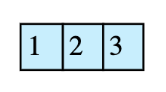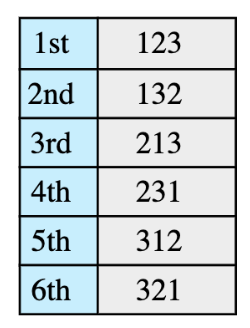# Find Kth Permutation

## Problem Statement

Given a set of ‘n’ elements, find their Kth permutation. Consider the following set of elements:All permutations of the above elements are (with ordering):Here we need to find the Kth permutation

• Recursion
• Factorial

## Try it yourself

void find_kth_permutation(    vector<char>& v,    int k,    string& result) {    //TODO: Write - Your - Code}

## Solution

int factorial(int n) {  if (n == 0 || n == 1) return 1;  return n * factorial(n -1 );}void find_kth_permutation(    vector<char>& v,    int k,    string& result) {  if (v.empty()) {    return;  }    int n = (int)(v.size());  // count is number of permutations starting with each digit  int count = factorial(n - 1);  int selected = (k - 1) / count;  result += v[selected];  v.erase(v.begin() + selected);  k = k - (count * selected);  find_kth_permutation(v, k, result);}string get_permutation(int n, int k) {    vector<char> v;    for (char i = 1; i <= n; ++i) {        v.push_back(i + '0');    }        string result;    find_kth_permutation(v, k, result);    return result;}int main(int argc, char* argv[]) {    for (int i = 1; i <= factorial(4); ++i) {        cout << i << "th permutation = " << get_permutation(4, i) << endl;    }}

## Solution Explanation

Linear, O(n).

### Memory Complexity

Linear, O(n).

Recursive solution will consume memory on the stack.

### Solution Breakdown

Here is the algorithm that we will follow:

If input vector is empty return result vector

block_size = (n-1)! ['n' is the size of vector]
Figure out which block k will lie in and select the first element of that block
(this can be done by doing (k-1)/block_size )

Append selected element to result vector and remove it from original input vector

Deduce from k the blocks that are skipped i.e k = k - selected*block_size and goto step 1


Practice problems like this and many more by checking out our Grokking the Coding Interview: Patterns for Coding Questions course!

Learn in-demand tech skills in half the time